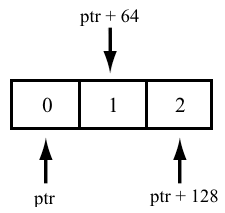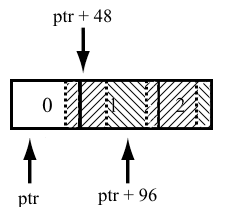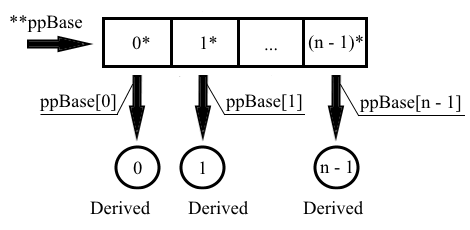﻿ V777. Dangerous widening type conversion from an array of derived-class objects to a base-class pointer.# V777. Dangerous widening type conversion from an array of derived-class objects to a base-class pointer.

The analyzer detected a possible error that has to do with accessing an array consisting of objects of a derived class by using a pointer to the base class. Attempting to access an element with a nonzero index through a pointer to the base class will result in an error.

Consider the following example:

``````class Base
{
int buf;

public:
virtual void Foo() { ... }
virtual ~Base() { }
};

class Derived : public Base
{
char buf;

public:
virtual void Foo() override { ... }
virtual ~Derived() { }
};

....
size_t n = 5;
Base *ptr = new Derived[n];   // <=
....
for (size_t i = 0; i < n; ++i)
(ptr + i)->Foo();
....``````

This code uses a base class "Base" and a class derived from it, "Derived". Each object of these classes occupies 48 and 64 bytes respectively (due to class alignment on an 8-byte boundary; the compiler used is MSVC, 64-bit). When "i >= 1", the pointer has to be offset by "i * 64" bytes each time when accessing an element with a nonzero index, but since the array is accessed through a pointer to the "Base" base class, the offset will actually be "i * 48" bytes.

This is how the pointer's offset was meant to be computed:This is how it is actually computed:In fact, the program starts handling objects containing random data.

This is the fixed code:

``````....
size_t n = 5;
Derived *ptr = new Derived[n];   // <=
....
for (size_t i = 0; i < n; ++i)
(ptr + i)->Foo();
....``````

It is also a mistake to cast a pointer that refers to the pointer to the derived class to a pointer that refers to the pointer to the base class:

``````....
Derived arr;
Derived *pDerived = arr;
Class5 **ppDerived = &pDerived;
....
Base **ppBase = (Derived**)ppDerived; // <=
....``````

To ensure that an array of derived-class objects is properly stored in a polymorphic way, the objects have to be arranged as shown below:This is what the correct version of this code should look like:

``````....
size_t n = 5;
Base **ppBase = new Base*[n]; // <=

for (size_t i = 0; i < n; ++i)
ppBase[i] = new Derived();
....``````

If you want to emphasize that you are going to handle one object only, use the following code:

``````....
Derived *derived = new Derived[n];
Base *base = &derived[i];
....``````

This code is considered safe by the analyzer and does not trigger a warning.

It is also considered a valid practice to use such a pointer to access an array consisting of a single object of the derived class.

``````....
Derived arr;
Derived *new_arr = new Derived;
Derived *malloc_arr = static_cast<Base*>(malloc(sizeof(Derived)));
....
Base *base = arr;
base = new_arr;
base = malloc_arr;
....``````

Note. If the base and derived classes are of the same size, it is valid to access an array of derived-class objects though a pointer to the base class. However, this practice is still not recommended for use.

 According to Common Weakness Enumeration, potential errors found by using this diagnostic are classified as CWE-468.

384
13 926

### Do you make errors in the code?